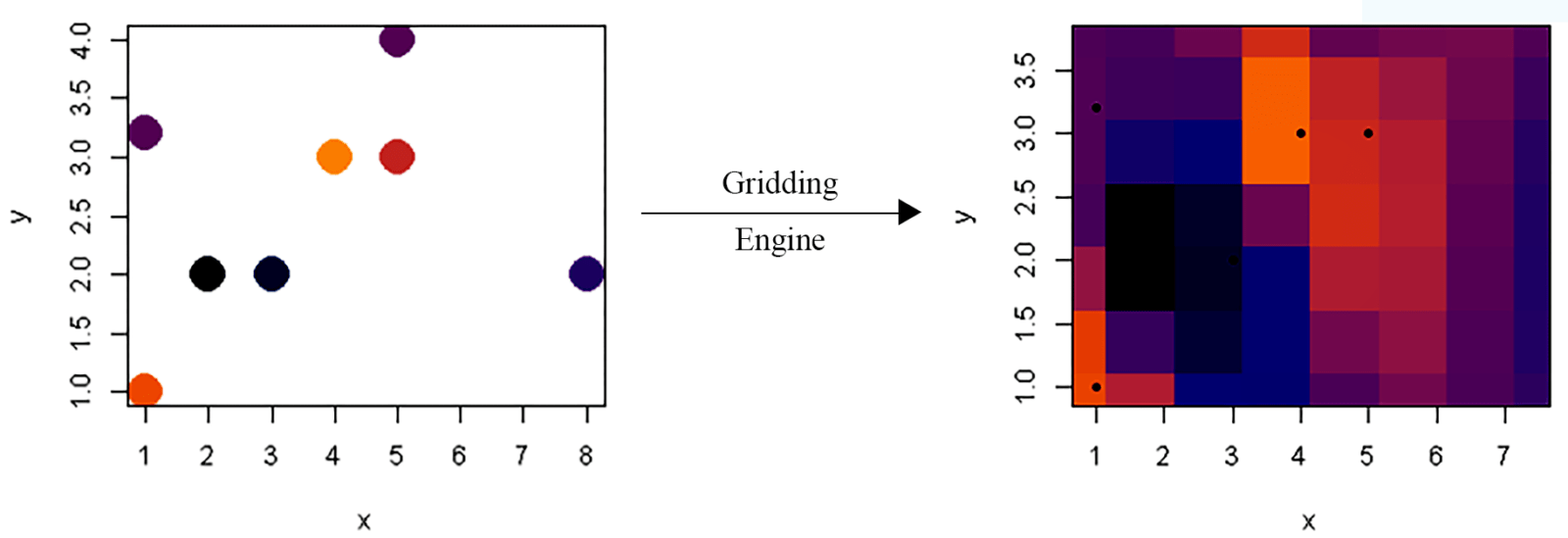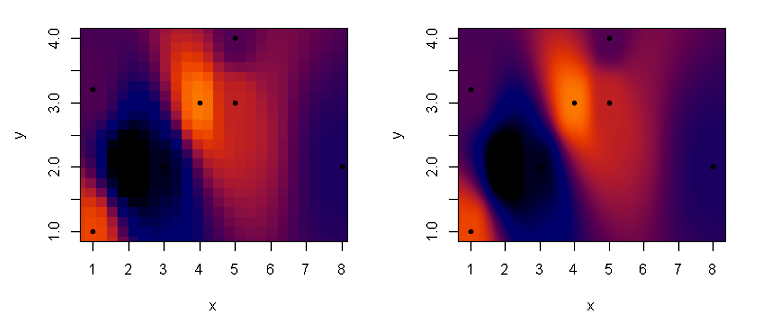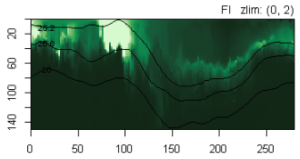[This article was first published on R Programming – Thomas Bryce Kelly, and kindly contributed to R-bloggers]. (You can report issue about the content on this page here)
Want to share your content on R-bloggers? click here if you have a blog, or here if you don't.

So all oceanographers are familiar with the results of gridding, even if they are not so familiar with the process. Gridding is, in general, any method that will take observations and output interpolated (and sometimes extrapolated) data that is placed onto a regular, well-behaving grid. Below is a simple illustration of just such a process where we take scattered observations of temperature and construct a very regular map of temperatures.Gridding can be done to arbitrary resolution (even though the accuracy does not increase!) at the expense of computational resources:Although oceanographers and other scientists may use the gridded data products of others regularly, many are unaware of the work that went into generating the gridded dataset from otherwise messy observations. And while the topic of gridding deserves multiple posts itself, today we’re only going to discussing some way of speeding up the gridding algorithm I wrote in R. For is an example of a section plot, a type of data gridding that is ubiquitous in chemical oceanography. The data was taken in the Arctic Ocean with depth on the y axis and latitude on the x. Actual sample locations  are denoted as black points.

[[ Example of a section plot from Laura ]]

The original gridding algorithm took X minutes to run, which is acceptable but slow. So my first step was to determine which part of the algorithm was the bottleneck. Without much thought I knew this would be costly part of the algorithm since it’s going entry by entry and calculating the value of the grid cell based on surrounding observations.

```for (i in 1:nrow(grid)) {

## Calculate distances and determine weights
del = delta(x.factor, y.factor, x, grid\$x[i], y, grid\$y[i])

w = W(del, delta.min) # Get weights
ll = order(w, decreasing = TRUE)[1:neighborhood]  # isolate to Neighborhood of n-closest values

w = w / sum(w[ll]) # Normalize
grid[[field.name]][i] = sum(z[ll] * w[ll])
}```

While my first thought was to parallelize this since the value of any particular grid cell does not rely on the values of any other cell–just the observations. This kind of problem is called “embarrassingly parallel” and promises nearly N-fold speedup on an N-core computer. Without going to the technical details I gave up on this approach since many parallel processing wrappers in R are platform specific and I needed code that readily worked for me and my collaborators.

Instead I opted to work on optimizing the existing code by vectorizing the process. Since the algorithm is embarrassingly parallel, R has several functions that allow you to make use of this information in order to speed up the calculation. A simple example of a vectorize procedure would be in adding up the columns of a matrix. Instead of going through each row and calculating  the sum of the entries in that row, we can tell the computer to take one column and add it to another column. The compiler, or virtual machine, now knows that it can perform this operation however it wants and in a more optimized way than just looping through matrix rows. Here’s the resulting code, which does the same procedure as the above code snippet:

`grid[[field.name]] = apply(grid, 1, function(row) {sum(z * W2(delta(x.factor, y.factor, x, row, y, row), delta.min))})`

Here is it the apply() function that performs the vectorized magic(1)NB: There is no such thing as magic, just advancement.. Furthermore, the speedup it gave was surprising: ~10x!

While there is certain to be many more optimization that I can do (including writing some of these functions in C or Fortran), for now the efficiency has reached a good point. Instead of waiting a minute for results, the algorithm finishes in just a few seconds with some figure-worthy results.As always, the code I use here is freely available under an MIT open source license.

Notes   [ + ]

 1 ↑ NB: There is no such thing as magic, just advancement.